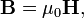# Coulomb's law (magnetic)

In physics, the magnetic Coulomb law is the magnetic equivalent of the electric Coulomb law. An important difference is that—at the present state of knowledge—"magnetic charges" (magnetic monopoles) do not exist in nature, whereas electric charges do exist. However, with the aid of a long bar magnet the force between the poles can be measured reasonably accurately.

The Coulomb force is inversely proportional to the squared distance between the magnetic poles Q1 and Q2, repulsive between like poles (N–N, S–S) and attractive between unlike poles (N–S).

Another difference with the electric Coulomb law is that in SI units there are two common measures of "amount of magnetism", i.e., the magnitudes of Q1 and Q2. This is due to the fact that there are two, closely related, vector fields giving the magnetic force. There is the magnetic field H and the magnetic induction B, which are related (in vacuum and in SI units) through$\mathbf{B} = \mu_0 \mathbf{H},$

where μ0 is the magnetic constant. The force between the poles of the magnets shown in the figure is the same, irrespective of the system of units used to express the North poles. We formulate the Coulomb force in two ways,$|\mathbf{F}| = \frac{Q_1' Q_2'}{4\pi \mu_0 r^2} = \frac{\mu_0 Q_1 Q_2}{4\pi r^2} \quad\Longrightarrow \quad Q_1' = \mu_0 Q_1,\; Q_2' = \mu_0 Q_2 . \qquad\qquad\qquad(1)$

If Q2 and Q'2 are infinitesimally small test "magnetic charges", which we divide out, we have the respective magnetic fields\begin{align} \mathbf{H} &= \frac{1}{\mu_0} \mathbf{B} \quad\hbox{with} \quad \mathbf{B} = \frac{Q_1'}{4\pi r^2}\\ \mathbf{B} &= \mu_0 \mathbf{H} \quad\hbox{with} \quad \mathbf{H} = \frac{Q_1}{4\pi r^2}\\ \end{align}

The unit of B is weber/m2 and hence the magnetic pole Q'1 is measured in weber. The unit of H is ampere⋅turn/m and hence the dimension of Q1 is ampere⋅turn⋅m.

In summary, there are two consistent SI formulations of Coulomb's law for magnetic poles, see Eq. (1). The magnetic poles in the two formulations differ by the magnetic constant μ0 and have different dimensions.